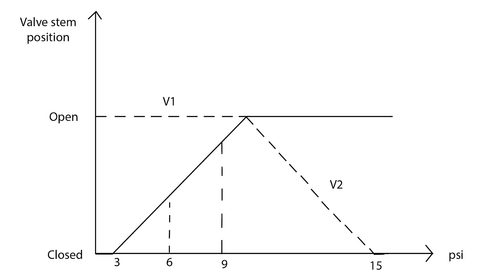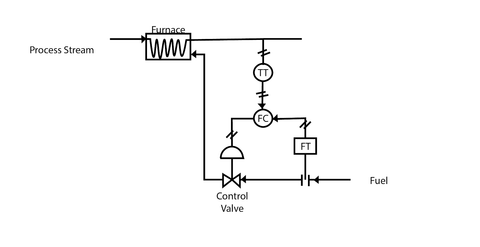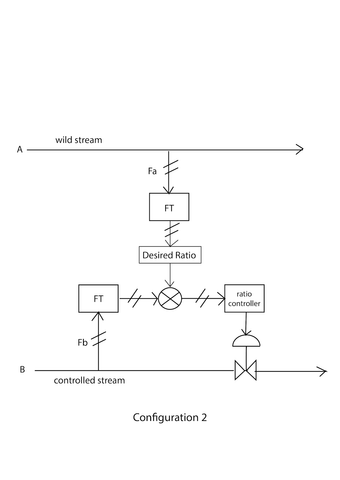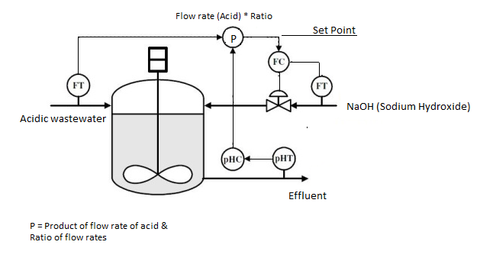# An Introduction To Process Control Schemes - Part II

In our Introduction To Process Control Schemes Part 1 blog, we talked about various jargon that you come across while studying control schemes and further discussed two different types of control schemes namely Feedback and Feedforward, looking at their applications in industries.

Today we will be understanding three different control schemes with their applications.

Beginning with Split Range Control Scheme,

## SPLIT RANGE CONTROL SCHEME

In the split range control configuration, we have one measured/process variable and several manipulated variables. Also, we have one measured signal, which is then divided into multiple parts and sent to affect all the available manipulated variables to achieve a perfect set point.Block diagram of Split Range control

In the block diagram, MV1 & MV2 are manipulated variables. There can be an ‘n’ number of manipulated variables.

Example of split range control## Split Range Control for Pressure Control of the Gas-Phase Reactor.

In the above diagram, the pressure of the reactor needs to be maintained at a constant value, and to keep the pressure at a constant value, there are two control valves placed at the inlet and outlet of the reactor. This means there are two manipulated variables in this example, being, the flow of reactants and products. The pressure of the reactor is measured using a pressure transmitter. This pressure is then transmitted to the controller. But in this example, the two control valves act in coordination to control the pressure of the reactor. Let the controller’s output signal be 6psig. And the setpoint is 9psig.  From the graph, we see that when the pressure is 6psig valve V1 is fully open while valve V2 is partly open and as the pressure increases to 9psig valve V2 opens continuously while V1 is fully open. As the pressure increases above this point valve V1 is completely closed and V2 is completely open.

You can understand the aforementioned valve operation from the following graph.Going by the definition of “Cascade” which is 'connecting the output of the first stage to the input of the next stage', the Cascade control scheme has two feedback loops where one feedback loop is inside the other. This control scheme helps improve the response to immeasurable disturbances, thus, reducing the errors drastically as compared to other control schemes.In a single loop feedback system, the error is calculated by comparing the output of the system to desired output and then passed to the controller which makes changes in the process variables to give the desired outcome. The Cascade system enhances the performance of this single-loop feedback system by using its multiloop structure and a secondary process following the primary process. The Cascade control system involves two controllers and the first controller decides the set point for the second one.

This system is effective only when the variable of the secondary process is faster than the primary process variable. The disturbances in the secondary loop are taken care of by the secondary controller before it affects the output and the primary controller takes care of other disturbances in the outer loop.

This, however, increases the cost of installation since it is more complex than a single loop control system. Also, tuning the cascade control system becomes quite difficult as the setpoint changes and parameters keep on increasing. To reduce the sensitivity and non-linearity of the system a strong secondary loop is needed which will reduce the parameter variations for the primary loop, thereby making the tuning of the cascade system a bit easy.

Note: the secondary loop should be at least three times faster than the primary loop to keep the system stable.

Explore Arduino Collection

## Cascade Control System for FurnaceOver here, the secondary loop is the one that will control the flow rate of the fuel while the primary loop is the one that controls the temperature of the furnace.

The temperature of the process stream is measured by the temperature transmitter in the primary control loop and the flow of the fuel is measured by the flow transmitter in the secondary control loop.

Any disturbances that occur in the fuel flow are corrected and flow is maintained at a constant value by the flow controller in the secondary loop and there will not be any effect on the output of the primary loop.

As a result, the temperature of the furnace will be maintained at a set point.

Check out Raspberry Pi Collection

## RATIO CONTROL SCHEME

It is a special type of feedforward control where two inputs entering a process are measured and held in a constant ratio against each other. It is mostly used to control the flow rates of the streams. Out of two streams only one stream can be controlled, the stream that is not controlled is called ‘wild stream’.

There are two configurations for implementing ratio control

### Configuration 1

In this configuration, the flow rates of both streams are measured. These measurements are then divided to obtain a ratio which is then compared to the setpoint ratio, and the deviation between them will produce an actuating signal for the control valve.### Configuration 2

In this configuration flow rate of the wild stream is measured and multiplied by the desired ratio. This results in a flow rate of the controlled stream. This value is then compared to the setpoint ratio and the resulting signal between them is used as an actuating signal for the control valve.An application of the Ratio control system is the process of neutralization of acidic wastewater by NaOH (sodium hydroxide). The flow of acidic wastewater will be measured but not changed therefore it is called a “wild stream”' and it is the uncontrolled variable in the process. So we have to control the flow of NaOH in the tank by maintaining the ratio of the flow rate of acidic wastewater to the flow rate of NaOH. The pH value of effluent is measured by the sensor and the pH controller sends the ratio of the flow rates which is to be maintained. The product of the measured flow rate of acidic wastewater and the ratio of flow rates gives us the set point for the flow rate of NaOH. The setpoint is the amount of NaOH which must be supplied in the tank to neutralize the solution. The flow controller actuates the control valve according to the setpoint thereby varying the flow of NaOH which neutralizes the solution. In this way treatment of acidic wastewater is carried out by a Ratio control scheme in Industries.This blog has been submitted by Cyborg, NIT Rourkela under the Robocraze Club Outreach Program.